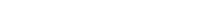# Math riddles Level 19 Answer with Hints and solutionsMath riddles game level 19 Answer with solution android game developed by Black games. Scroll Below to find details.

Math Riddles tests your IQ with mathematical puzzles. Challenge yourself with different levels of math puzzles and stretch the limits of your intelligence. Every IQ game is prepared with an approach of an IQ test. Improve your mathematics, challenge your brain with this game. There are many hard levels which you may not have solved then you can take help from here.

Math app level 19:Solution: power 0= 1

If there is any Doubt/discrepancy then please let me know in comments or you are unable to understand solution then I will surely help you.

### 11 thoughts on “Math Riddles App Level 19 Solution Android”

•September 21, 2019 at 6:21 am

First you must remember that every number squared to 0 equals 1 It is a mathematical law!!!
Try to write down the equation like this:
(1^5) +[(5^2) / (25^0)] -(5^1) =
1+ (25/1) -5 =
1+25-5 = 26-5 = 21
or: 1 + 20 = 21

•September 1, 2019 at 3:36 am

I bet you meant 25

•June 23, 2019 at 9:56 am

Hello

•May 29, 2019 at 8:57 pm

1^5+5^2/25^0-5^1=
1+25/1-5
1+25-5
26-5=21
RESPUESTA: 21

•April 21, 2019 at 12:31 pm

Your math is wrong. I’m honestly not even sure how you got 21 as an answer to begin with.

For those reading this that don’t know, the “^” symbol means “to the power of” symbolizing an exponent ie: 1^5 means 1 to the power of 5 or 1x1x1x1x1. order of operations is as follows: parenthesis, exponents, multiply, divide, add, subtract and is always to be solved from left to right. a simple way to remember is Please Excuses My Dear Aunt Sally.

1^5+5^2/25^0-5^1=x
1+5^2/25^0-5^1=x
1+25/25^0-5^1=x
1+25/1-5=x
26/4+x
6.5=x

this is how the equation should have been solved. please fix this

•May 23, 2019 at 4:45 am

No, perché prima di risolve la divisione, e poi l’addizione e la sottrazione!

•June 4, 2019 at 11:28 pm

This!!
Though, it should be – 6.5 as 1 – 5 = – 4

•June 20, 2019 at 2:37 am

25^0 is not 1
it’s just 0

•September 21, 2019 at 6:27 am

Division and Multiplication are always done first. 1+(25/1) -5 = 1 +25 -5 = 21

•March 17, 2019 at 8:59 am

Mohon penjelasannya.

•April 17, 2019 at 5:08 am